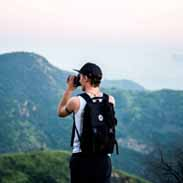# 3rd Grade – Newton’s 3 Laws of Motion and Scientific MethodRoy Johnson

## Unlock all answers in this set

question
What is GRAVITY?
GRAVITY is the force that pulls things down to the ground.
question
What is FORCE?
A FORCE is always a push or a pull.
question
Why did the paperclip attached to the parachute fall more slowly than the plain paper clip?
Because of a force called AIR RESISTANCE. (The opposition towards the motion of a body by the air)
question
What is FRICTION?
FRICTION is the force that slow things down or stops them.
question
What is Isaac Newton's 1st Law of Motion?
An object in motion stay in motion and an object at rest stays at rest unless acted upon by an outside force.
question
Give an example of Newton's 1st Law?
A soccer ball sitting on a field will stay there until something comes along to move it like the wind or a kick.
question
What is Isaac Newton's 2nd Law of Motion?
The weight of an object does not have an effect on the speed with which it falls. (All objects fall at the same speed)
question
What is Isaac Newton's 3rd Law of Motion?
For every action there is an equal and opposite reaction.
question
Give an example of Newton's 3rd Law?
The experiment with the "balloon jets" proved Newton's 3rd Law when the balloon with the most air traveled the furthest.
question
Which one of Newton's Laws of Motion did the "CARD SHARKS" lab demonstrated?
Newton's 1st Law
question
Which one of Newton's Laws of Motion did the "BALLOON JETS" demonstrate?
Newton's 3rd Law
question
Which one of Newton's Laws of Motion did the "LOOK OUT BELOW" lab demonstrate?
Newton's 2nd Law
question
What is the force that pulls things down to the ground called?
Gravity
question
What is the force that slows things down or stops them called?
Friction
question
What force causes the paper clip attached to the parachute to fall more slowly than the plain paper clip?
Air resistance
question
List the 5 steps of the scientific method?
Queen Penelope picks out clothes. Q = Question P = Prediction P = Procedure O = Observation C = Conclusion
question
What is the first step of the scientific method called?
Question
question
What is a hypothesis?
An educated guess.
question
What is the part of the scientific method called where you form a hypothesis?
Prediction. It is the second step.
question
What is the part of the scientific method called where you tell what you did?
Procedure
question
What is the part of the scientific method called where you take notes and record information?
Observation
question
What is the part of the scientific method called where you explain why something happened?
Conclusion
question
A book left on the table overnight is there when you return in the morning. This is an example of which Newton's Law?
1st
question
A space shuttle propels gas particles out the back of the shuttle and is able to travel forward through space. This is an example of which Newton's Law?
3rd
question
You drop a ping-pong ball and a golf ball. Both hit the floor at the same time. This is an example of which Newton's Law?
2nd
question
A balloon flies forward when air quickly escapes from it. This is an example of which Newton's Law?
3rd
question
A soccer ball continues to roll until it hits something. Which Law is it?
1st
question
The clothes you left on the floor are still there when you come home from school. Which Law is it?
1st
question
You and your dad jump off a wooden box and land at the same time. Which Law is it?
2nd
question
The bike you are riding hits a hole and stops quickly, but you fly forward over the handlebars. Which Law is it?
1st
question
Predict what motion will happen according to Newton's 1st Law: If you leave a cookie on a plate and there is no one else in the house, where will the cookie be in an hour?
Still on the plate
question
Predict what motion will happen according to Newton's 1st Law: If you were in outer space and threw a baseball, what would happen?
It would stay in motion
question
Predict what motion will happen according to Newton's 2nd Law: If your little brother drops a book and a cardboard box at the same time, what will happen?